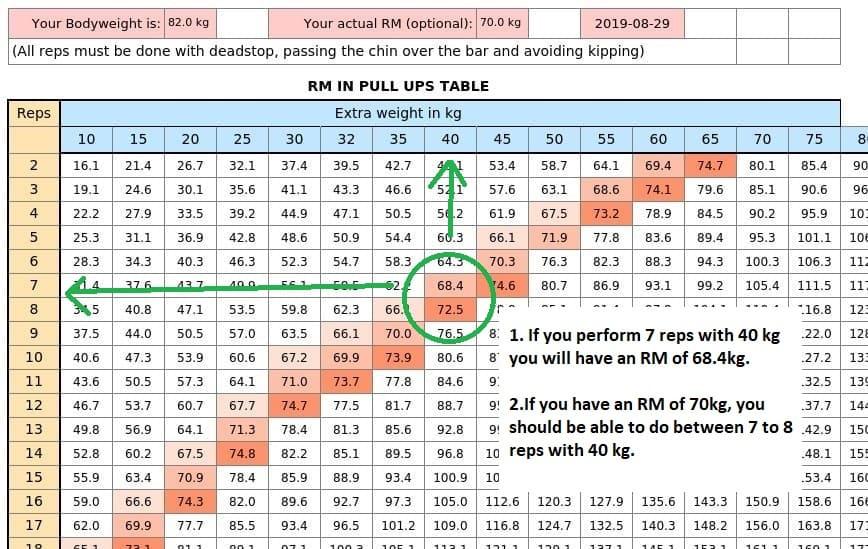## Max Bench Calculator

Unit weight type:

#### Option 1: Compute specific result

Calculator Mode :

Number of Repetitions :

#### Option 2: Compute the table

Unit weight type:

Your RM (repetition max) is :

This is the One Repetition Max Calculator for Bench Press.

The bench calculator has two options, the first one for specific results and the second to compute a full one repetition max table.

The first option will allow to compute your 1RM specifying the number of repetitions with a certain weight. The way it works is very easy: You just need to input the added weight in the first box and the number of repetitions in the following box. The calculator will automatically estimate your one rep max.

There is one consideration for estimating your 1RM which is that the lower the number of repetitions, the more accurate the result will be. You have to understand that the calculator just returns an estimation of your 1RM, and the further you are from your maximum the less accurate will be.

This one rep max Bench Calculator has some other modes, that you can find in the dropdown list. They allow to compute the other variables (the repetitions or the added weight) for a specific Maximum Repetition. They work by just isolating the one repetition max equation.

Compute the one repetition max table blow.

### What will you obtain?

This calculator returns a table with added weight in the horizontal axis and number of repetitions in the vertical axis. In the cells inside the table you will see a number. That number is the 1RM that you could do for the given Reps and Added weight. See the example below.